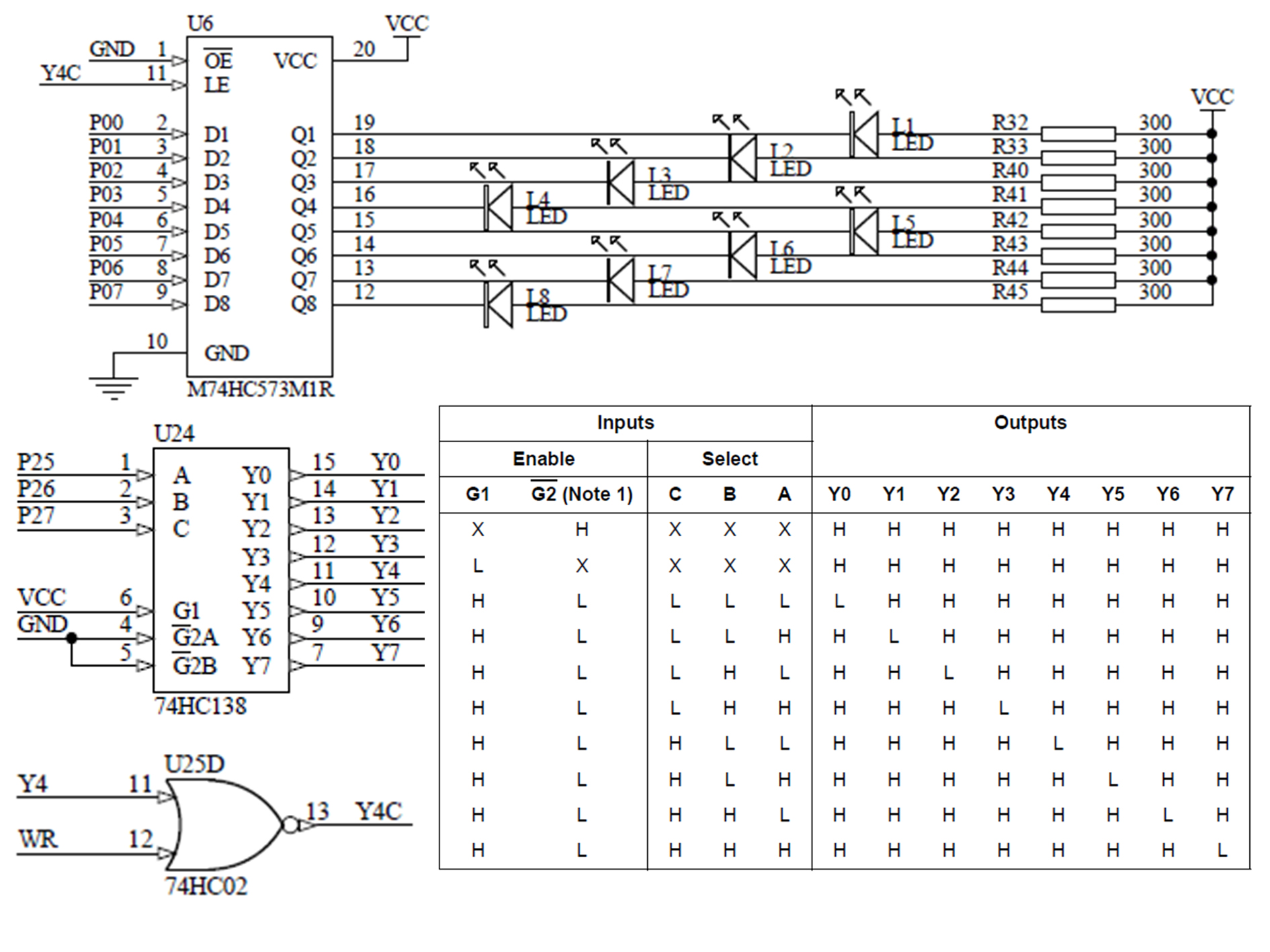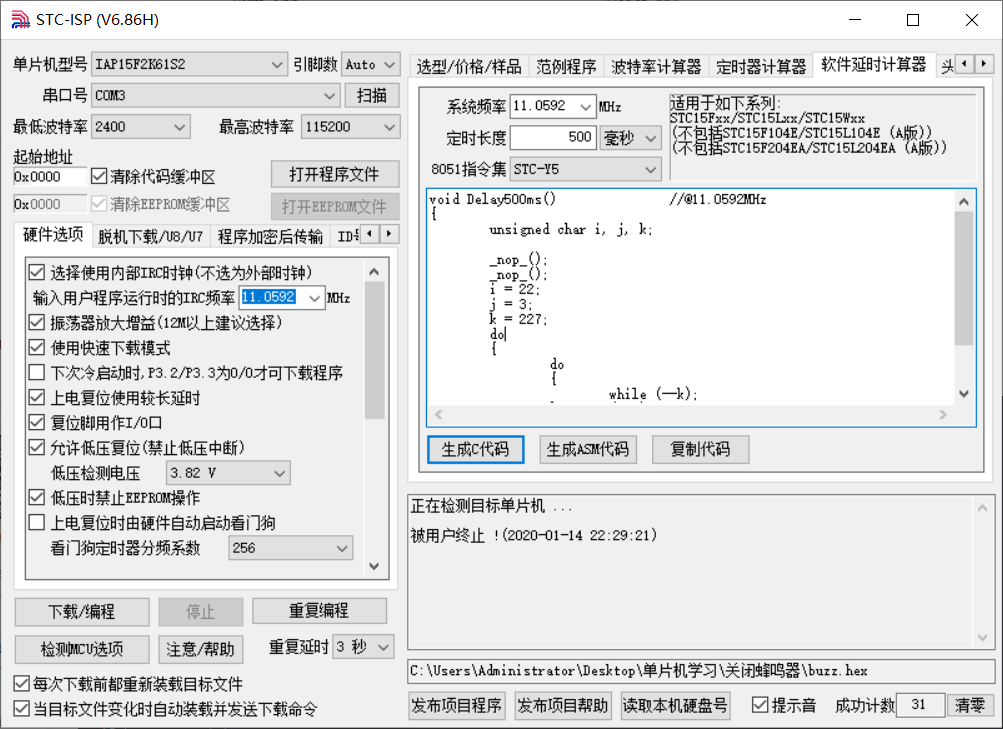# 蓝桥杯——流水灯

## 一、原理部分### 延时函数的获取## 二、代码实现

#include "stc15f2k60s2.h"
#include "intrins.h"
sbit buzz = P0^6;		 //位定义蜂鸣器
unsigned char a = 0x80;	 //使用a来进行移位操作

void Delay500ms()		//延时0.5ms函数
{
unsigned char i, j, k;

_nop_();
_nop_();
i = 22;
j = 3;
k = 227;
do
{
do
{
while (--k);
} while (--j);
} while (--i);
}

void close_buzz()	//蜂鸣器上电默认高电平，所以关闭蜂鸣器
{
P2=0xa0;
buzz = 0;
P2=0x00;
}

void main()
{
close_buzz();
while(1)
{
P2=0x80;
a = _crol_(a,1);
P0 = a;
P0 = ~P0  ;
P2=0x00;
Delay500ms();
}
}



©️2019 CSDN 皮肤主题: 大白 设计师: CSDN官方博客